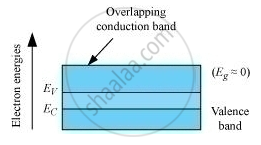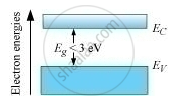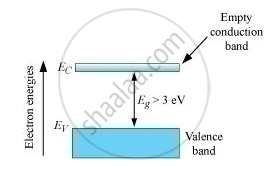PUC Karnataka Science Class 12Department of Pre-University Education, Karnataka
Share

# Distinguish Between a Conductor, a Semiconductor and an Insulator on the Basis of Energy Band Diagrams. - PUC Karnataka Science Class 12 - Physics

ConceptEnergy Bands in Conductors, Semiconductors and Insulators

#### Question

Distinguish between a conductor, a semiconductor and an insulator on the basis of energy band diagrams.

#### Solution

The energy-band diagram of a conductor is represented asThe energy-band diagram of a semiconductor is represented asThe energy-band diagram of an insulator is represented asIs there an error in this question or solution?

#### Video TutorialsVIEW ALL 

Solution Distinguish Between a Conductor, a Semiconductor and an Insulator on the Basis of Energy Band Diagrams. Concept: Energy Bands in Conductors, Semiconductors and Insulators.
S